# Largest prime divisor

This article defines an arithmetic function or number-theoretic function: a function from the natural numbers to a ring (usually, the ring of integers, rational numbers, real numbers, or complex numbers).
View a complete list of arithmetic functions

## Definition

Letbe a natural number greater than. The largest prime divisor ofis defined as the largest among the primesthat divide. This is denoted as. By fiat, we set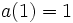.

For a positive real number, we say thatis a-smooth number if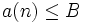. Otherwise,is a-rough number.

## Behavior

The ID of the sequence in the Online Encyclopedia of Integer Sequences is A006530

### Initial values

The initial values of the largest prime divisor are: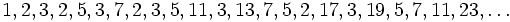.

### Lower bound

There are infinitely many powers of two, and hence,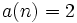for infinitely many numbers and this is the best lower bound.

### Density results

Further information: Dickman-de Bruijn function

• For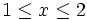, the density of numberssuch thatis given by. Hence the density of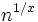-smooth numbers is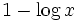.
• For general, the density of numberssuch thatis still positive. This density (or rather, the density of the complement) is described by the Dickman-de Bruijn function, which occurs as the solution to a delay differential equation.